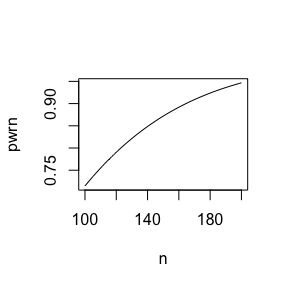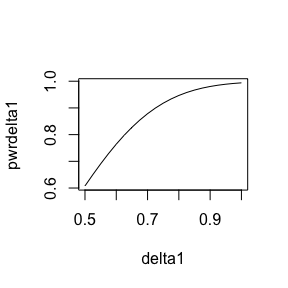# Introduction

Limited support is provided for 2-sample design with a normally distributed random variable as the outcome. Users are encouraged to look at guidance such as in Jennison and Turnbull (2000). We provide a tool where for a large sample case where a reasonable estimate of standard deviation is available, a reasonable sample size can be computed based straightforward distribution theory outlined below.

# The problem considered

The overall sample size notation used for gsDesign is to consider a standardized effect size parameter which is referred to as $$\theta$$ in Jennison and Turnbull (2000). We begin with the 2-sample normal problem where we assume a possibly different standard deviation in each treatment group. For $$j=1,2$$, we let $$X_{j,i}$$, $$i=1,2,\ldots n_j$$ represent independent and identically distributed observations following a normal distribution with mean $$\mu_j$$ and standard deviation $$\sigma_j$$. The natural parameter for comparing the two distributions is

$\delta = \mu_2 - \mu_1$

and we wish to test if $$\delta > 0$$ in a one-sided testing scenario to test for superiority of treatment 2 over treatment 1. We could also consider testing, say, $$\delta > \delta_0$$ for a non-inferiority scenario with $$\delta_0<0$$ or super superiority if $$\delta_0>0$$. While normally a t-test would be used for this, for large sample sizes this would be nearly equivalent to a Z-test defined by:

$Z=\frac{\bar X_2 - \bar X_1-\delta_0}{\sqrt{\sigma^2_2/n_2 + \sigma_1^2/n_1}}\approx \frac{\bar X_2 - \bar X_1}{\sqrt{s^2_2/n_2 + s_1^2/n_1}}=t$ where $$\bar X_j$$ is the sample mean and $$s_j^2$$ is the sample variance for group $$j=1,2$$. The far right hand side of this is Welch’s t-test. For our examples we use this $$t$$-test and show that the sample size computation based on the $$Z$$-test above works well for the chosen problems.

# Sample size

Thus, $$n_2=rn/(1+r)$$, $$n_1=n/(1+r)$$ and when $$r=1$$ we have $$n_1=n_2=n/2$$. Now that we have completed needed notation, those not interested in the theory behind the sample size and power calculation used may skip the rest of this section.

We let $\sigma^2=(1+r)(\sigma_1^2+\sigma_2^2/r)$ and define $\theta= (\delta -\delta_0)/\sigma.$ Under the given assumptions, $Z \sim \hbox{Normal}\left(\sqrt n\theta,1\right).$ Under the null hypothesis that $$\delta=\delta_0$$, we have $$Z\sim \hbox{Normal}(0,1)$$. Thus, regardless of $$n$$ we have $P_0[Z\ge \Phi^{-1}(1-\alpha)]=\alpha.$ Under the alternate hypothesis that $$\delta=\delta_1$$ and we denote a corresponding $$\theta_1$$. We define the type 2 error $$\beta$$ and power $$1-\beta$$ by

\begin{align} 1-\beta =& P_1[Z\ge \Phi^{-1}(1-\alpha)]\\ =& P[Z-\sqrt n\theta_1\ge \Phi^{-1}(1-\alpha)-\sqrt n\theta_1]\\ =&\Phi(\Phi^{-1}(1-\alpha)-\sqrt n\theta_1)). \end{align}

If the power $$1-\beta$$ is fixed, we can invert this formula to compute sample size with:

$n= \left(\frac{\Phi^{-1}(1-\beta)+\Phi^{-1}(1-\alpha)}{\theta_1}\right)^2.$

For 2-sided testing, we simply substitute $$\alpha/2$$ for $$\alpha$$ in the above two formulas.

# Examples

We consider two examples to check the above formulas vs. nNormal(). We then confirm that the approximation is working well by simulating and confirming that the power and Type I error approximations are useful. Finally, we provide a simple group sequential design example.

## Sample size

We consider an example with $$\sigma_2=1.25$$, $$\sigma_1=1.6$$, $$\delta=0.8$$ and $$\delta_0=0$$. We let the sample size ratio be 2 experimental group observations per control observation. We compute sample size with nNormal() assuming one-sided Type I error $$\alpha=0.025$$ and 90% power ($$1-\beta=0.9$$).

Checking using the sample size formula above, we have:

r <- 2
sigma <- sqrt((1+r)*(1.6^2 + 1.25^2/r))
theta <- 0.8/sigma
((qnorm(.9)+qnorm(.975))/theta)^2
#>  164.5684

## Power

Now, assume we let the sample size be 200 and compute power under the same scenario.

nNormal(delta1=0.8, sd = 1.6, sd2 = 1.25, alpha = 0.025, n = 200, ratio = 2)
#>  0.9466825

From the power formula above, we duplicate this with:

pwr <- pnorm(qnorm(.975) - sqrt(200) * theta, lower.tail = FALSE)
pwr
#>  0.9466825

If we want to plot power for a variety of sample sizes, we can input n as a vector:

n <- 100:200
pwrn <- nNormal(delta1 = 0.8, sd = 1.6, sd2 = 1.25, alpha = 0.025, n = n, ratio = 2)
plot(n, pwrn, type="l")Alternatively, you could fix sample size at 200 and plot power under different treatment effect assumptions:

delta1 <- seq(.5,1,.025)
pwrdelta1 <- nNormal(delta1 = delta1, sd = 1.6, sd2 = 1.25, alpha = 0.025, n = 200, ratio = 2)
plot(delta1, pwrdelta1, type="l")## Verification with simulation

Rather than simulate individual observations, we will take advantage of the fact that for $$j=1,2$$

$\bar X_j\sim \hbox{Normal}(\mu_j,\sigma_j^2/n_j)$ and

$(n_j-1)s_j^2/\sigma_j^2=\sum_{i=1}^{n_j} (X_{ij}-\bar X_j)/\sigma^2 \sim \chi ^2_{n_j-1}$

are independent. Thus, we can simulate trial power with $$n=200$$ 1 million times with a t-statistic with unequal variances quickly as follows under the alternate hypothesis:

nsim <- 1000000
delta <- 0.8
sd1 <- 1.6
sd2 <- 1.25
n1 <- 67
n2 <- 133
deltahat <- rnorm(n=nsim, mean=delta, sd=sd1/sqrt(n1)) - rnorm(n=nsim, mean=0, sd=sd2/sqrt(n2))
s <- sqrt(sd1^2 * rchisq(n=nsim, df=n1 - 1)/(n1-1)/n1 +
sd2^2 * rchisq(n=nsim, df=n2 - 1)/(n2-1)/n2)
z <- deltahat / s
mean(z >= qnorm(.975))
#>  0.946126

The standard error for this simulation power calculation is approximately

sqrt(pwr*(1-pwr)/nsim)
#>  0.0002246659

suggesting we should be within less than about 0.001 if the actual power, which suggests the normal power approximation is reasonable for this scenario.

## Group sequential design

Now we derive a group sequential design under the above scenario. We will largely use default parameters and show two methods. For the first, we plug in the fixed sample size above as follows:

d <-
gsDesign(k=2,
n.fix = nNormal(delta1=0.8, sd = 1.6, sd2 = 1.25, alpha = 0.025, beta = .1, ratio = 2),
delta1 = 0.8)
d %>%  gsBoundSummary(deltaname = "Mean difference") %>%
kable(row.names = FALSE)
Analysis Value Efficacy Futility
IA 1: 50% Z 2.7500 0.4122
N: 86 p (1-sided) 0.0030 0.3401
~Mean difference at bound 0.9399 0.1409
P(Cross) if Mean difference=0 0.0030 0.6599
P(Cross) if Mean difference=0.8 0.3412 0.0269
Final Z 1.9811 1.9811
N: 172 p (1-sided) 0.0238 0.0238
~Mean difference at bound 0.4788 0.4788
P(Cross) if Mean difference=0 0.0239 0.9761
P(Cross) if Mean difference=0.8 0.9000 0.1000

A textual summary of the design is given by:

cat(summary(d))

Asymmetric two-sided group sequential design with non-binding futility bound, 2 analyses, sample size 172, 90 percent power, 2.5 percent (1-sided) Type I error. Efficacy bounds derived using a Hwang-Shih-DeCani spending function with gamma = -4. Futility bounds derived using a Hwang-Shih-DeCani spending function with gamma = -2.

We can get the same answer by plugging in the standardized effect size we computed above:

gsDesign(k=2,
delta = theta,
delta1 = 0.8) %>%
gsBoundSummary(deltaname = "Mean difference") %>%
kable(row.names=FALSE)
Analysis Value Efficacy Futility
IA 1: 50% Z 2.7500 0.4122
N: 86 p (1-sided) 0.0030 0.3401
~Mean difference at bound 0.9399 0.1409
P(Cross) if Mean difference=0 0.0030 0.6599
P(Cross) if Mean difference=0.8 0.3412 0.0269
Final Z 1.9811 1.9811
N: 172 p (1-sided) 0.0238 0.0238
~Mean difference at bound 0.4788 0.4788
P(Cross) if Mean difference=0 0.0239 0.9761
P(Cross) if Mean difference=0.8 0.9000 0.1000

We leave it to the reader to verify the properties of the above design using simulation as in the fixed design example.